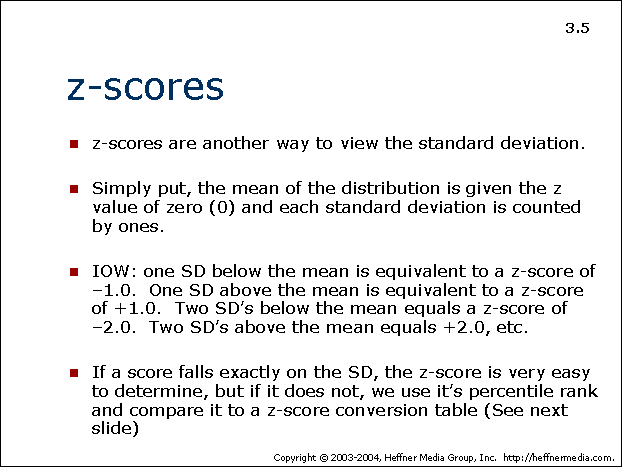advertisement

# 05## z-scores

• z-scores are another way to view the standard deviation.
• Simply put, the mean of the distribution is given the z value of zero (0) and each standard deviation is counted by ones.
• IOW: one SD below the mean is equivalent to a z-score of -1.0. One SD above the mean is equivalent to a z-score of +1.0. Two SD’s below the mean equals a z-score of -2.0. Two SD’s above the mean equals +2.0, etc.
• If a score falls exactly on the SD, the z-score is very easy to determine, but if it does not, we use it’s percentile rank and compare it to a z-score conversion table (See next slide)